# extrude

Vertically extrude 2-D geometry or specified faces of 3-D geometry

## Syntax

``extrude(g,height)``
``extrude(g,FaceID,height)``
``h = extrude(___)``

## Description

example

````extrude(g,height)` creates a 3-D discrete geometry by extruding a 2-D geometry along the z-axis by the value of `height`. You can create a stacked multilayered 3-D discrete geometry by specifying `height` as a vector of thicknesses of the layers.```

example

````extrude(g,FaceID,height)` extrudes specified faces of a 3-D geometry along the direction normal to the faces. Here, `FaceID` specifies which faces to extrude. You can extrude faces into multiple layers by specifying `height` as a vector of thicknesses of the layers.All of the specified faces must be flat and have the same orientation. The extruded volumes must not intersect with each other or with the existing geometry.```
````h = extrude(___)` returns a handle `h`. If the original geometry is a `DiscreteGeometry` object, then the function modifies the original geometry, and `h` a handle to the modified `DiscreteGeometry` object. If the original geometry is an `AnalyticGeometry` object, then `h` is a handle to a new `DiscreteGeometry` object. In this case, the original geometry remains unchanged.```

## Examples

collapse all

Create a 3-D geometry by extruding a 2-D geometry along the z-axis.

Create a PDE model.

`model = createpde;`

Import a 2-D geometry.

`g = importGeometry(model,'PlateHolePlanar.stl');`

Plot the geometry and display the face labels.

`pdegplot(g,'FaceLabels','on')`Create a 3-D geometry by extruding the 2-D geometry along the z-axis by 5 units.

`extrude(g,5)`
```ans = DiscreteGeometry with properties: NumCells: 1 NumFaces: 7 NumEdges: 15 NumVertices: 10 Vertices: [10x3 double] ```

Plot the new geometry and display the face labels.

`pdegplot(g,'FaceLabels','on','FaceAlpha',0.5)`Create a stacked multilayered 3-D geometry by extruding a 2-D geometry along the z-axis.

Create a PDE model.

`model = createpde;`

Import a geometry.

`g = importGeometry(model,'PlateHolePlanar.stl')`
```g = DiscreteGeometry with properties: NumCells: 0 NumFaces: 1 NumEdges: 5 NumVertices: 5 Vertices: [5x3 double] ```

Plot the geometry and display the face labels.

`pdegplot(g,'FaceLabels','on')`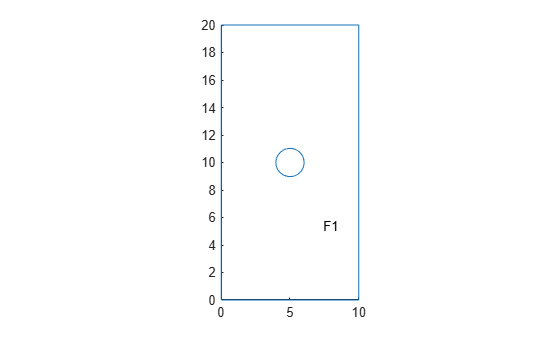Create a 3-D geometry consisting of three blocks with holes stacked on top of each other. The heights of the blocks are 5, 10, and 20 units.

`extrude(g,[5,10,20])`
```ans = DiscreteGeometry with properties: NumCells: 3 NumFaces: 19 NumEdges: 35 NumVertices: 20 Vertices: [20x3 double] ```

Plot the new geometry and display the cell labels.

`pdegplot(g,'CellLabels','on','FaceAlpha',0.5)`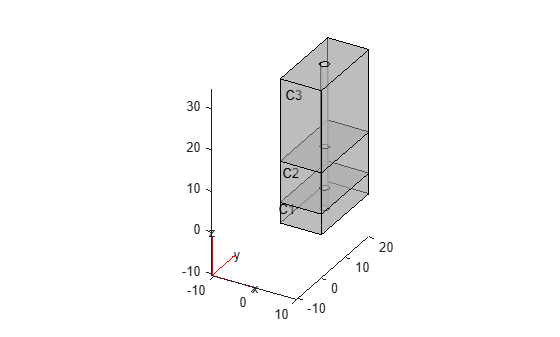Extrude a 2-D geometry that has a vertex added by the `addVertex` function. The layers of the extruded geometry all have a corresponding vertex, but there are no edges between these vertices.

Create a PDE model.

`model = createpde;`

Import a geometry.

`g = importGeometry(model,'PlateHolePlanar.stl');`

Plot the geometry and display the vertex labels.

`pdegplot(g,'VertexLabels','on')`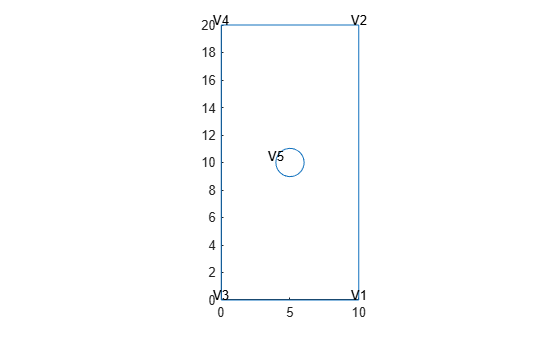Add a new vertex on the right edge.

`addVertex(g,'Coordinates',[10 12]);`

Plot the new geometry and display the vertex labels.

`pdegplot(g,'FaceLabels','on','VertexLabels','on')`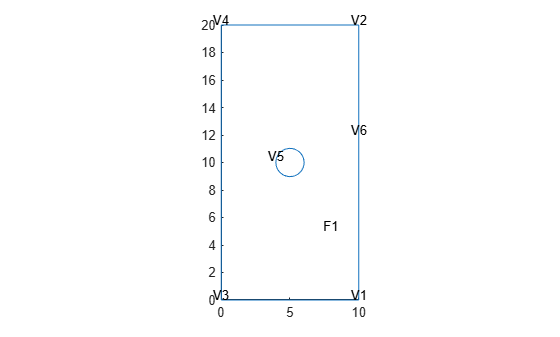Create a 3-D geometry consisting of three blocks with holes stacked on top of each other. The heights of the blocks are 5, 10, and 20 units.

`extrude(g,[5,10,20])`
```ans = DiscreteGeometry with properties: NumCells: 3 NumFaces: 19 NumEdges: 35 NumVertices: 24 Vertices: [24x3 double] ```

Plot the new geometry and display the vertex labels. The `extrude` function replicates the added vertex `V6` into three new vertices: `V12`, `V18`, and `V24`. It does not create edges between these vertices.

`pdegplot(g,'VertexLabels','on','FaceAlpha',0.5)`Extrude a 2-D geometry that has a face added by the `addFace` function.

Create a PDE model.

`model = createpde;`

Import a geometry.

`g = importGeometry(model,'PlateHolePlanar.stl');`

Plot the geometry and display the face and edge labels.

`pdegplot(g,'FaceLabels','on','EdgeLabels','on')`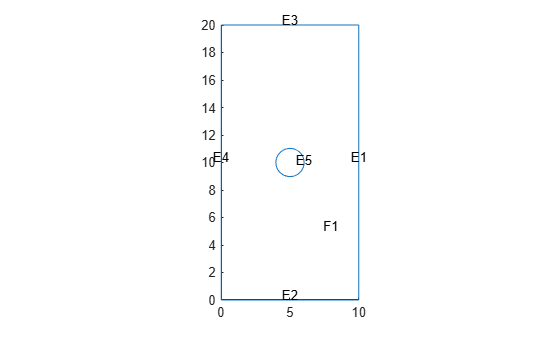Fill the hole in the center by adding a face.

`addFace(g,5)`
```ans = DiscreteGeometry with properties: NumCells: 0 NumFaces: 2 NumEdges: 5 NumVertices: 5 Vertices: [5x3 double] ```

Plot the modified geometry.

`pdegplot(g,'FaceLabels','on')`Create a 3-D geometry by extruding the 2-D geometry along the z-axis by 2 units.

`extrude(g,2)`
```ans = DiscreteGeometry with properties: NumCells: 2 NumFaces: 9 NumEdges: 15 NumVertices: 10 Vertices: [10x3 double] ```

Plot the new geometry and display the cell labels.

`pdegplot(g,'CellLabels','on','FaceAlpha',0.5)`Extrude specified faces of a 3-D geometry.

Import the geometry and plot it with the face and edge labels.

```g = importGeometry('PlateHolePlanar.stl'); pdegplot(g,'FaceLabels','on','EdgeLabels','on')```Fill the hole in the center by adding a face. Plot the modified geometry.

```addFace(g,5); pdegplot(g,'FaceLabels','on')```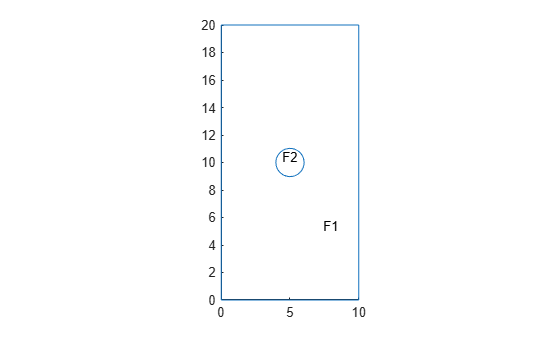Create a 3-D geometry by extruding the 2-D geometry along the z-axis by 2 units.

`extrude(g,2);`

Plot the new geometry with the cell and face labels.

`pdegplot(g,'CellLabels','on','Facelabels','on','FaceAlpha',0.5)`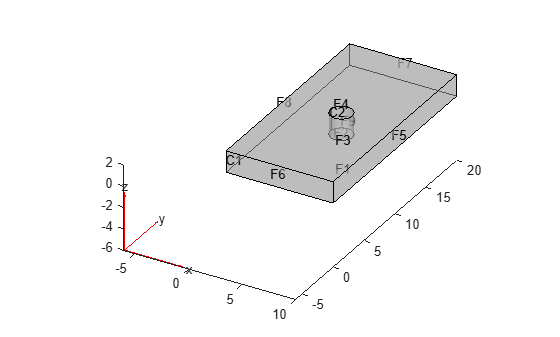Now, extrude the center face of the geometry by 5 units.

`extrude(g,4,5);`

Plot the resulting geometry with the cell labels.

`pdegplot(g,'CellLabels','on','FaceAlpha',0.5)`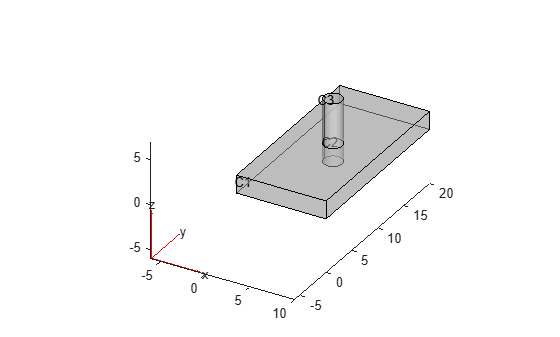Now, call `extrude` again, and this time specify a vector of heights. The function extrudes all specified faces by each of the specified heights, which creates multiple layers.

```extrude(g,[1 2],[3 4]); pdegplot(g,'CellLabels','on','FaceAlpha',0.5)```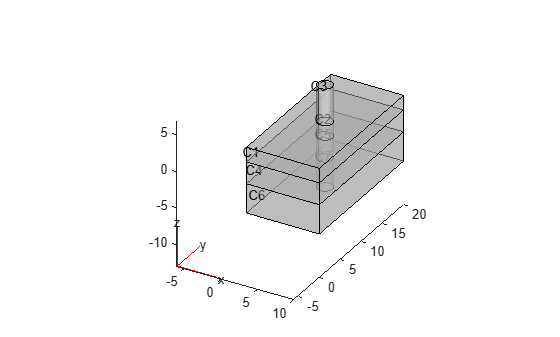## Input Arguments

collapse all

Geometry, specified as a `DiscreteGeometry` or `AnalyticGeometry` object.

Cell heights, specified as a positive real number or a vector of positive real numbers.

If `height` is a vector and `g` is a 2-D geometry, then `height(i)` specifies the height of the `i`th layer of a multilayered (stacked) 3-D geometry. Each layer constitutes a new cell.

If `g` is a 3-D geometry, the function extrudes all specified faces into several layers, with `height(i)` specifying the height of the `i`th layer.

Example: `extrude(g,5.5)`

Faces to extrude in 3-D geometry, specified as a positive real number or a vector of positive real numbers. If `height` is a vector, then the function extrudes all specified faces into several layers, same as it does for 2-D geometries.

## Output Arguments

collapse all

Resulting geometry, returned as a handle. If the original geometry `g` is a `DiscreteGeometry` object, then `h` is a handle to the modified `DiscreteGeometry` object `g`. If `g` is an `AnalyticGeometry` object, then `h` is a handle to a new `DiscreteGeometry` object. In this case, the original geometry `g` remains unchanged.

## Tips

• `extrude` modifies a geometry, but it does not modify the corresponding mesh. After modifying a geometry, regenerate the mesh to ensure a proper mesh association with the new geometry.

• If a 2-D geometry has new vertices added by using the `addVertex` function, `extrude` replicates the new vertices on each new layer of the extruded 3-D geometry, but it does not connect these vertices by edges.

• If `g` is an `AnalyticGeometry` object, and you want to replace it with the extruded discrete 3-D geometry, assign the output to the original geometry, for example, `g = extrude(g,20)`.

Introduced in R2020b

## Support#### Try MATLAB, Simulink, and Other Products

Get trial now